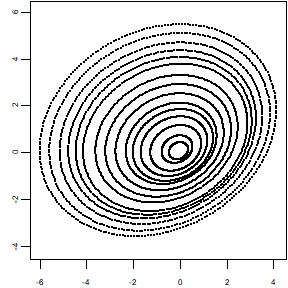# Simulating corneal datasets

The function simulateData permits to simulate a wide variety of datasets that appear in clinical practice, as a result of measuring an individual eye with a Placido-disk corneal topographer.

This function produces a dataset in the same format as the one read by readFile from a file, i.e., a list with three columns (x and y coordinates of each point and its ring index) and a row per data point, according to the function parameters (by default, 6144 rows or data points).

The following examples show different ways of using simulateData, by adding different transformations or perturbations to the basic circular pattern. Some of the obtained patterns can correlate with certain clinical conditions, such as keratoconus, comma, or others.

## Example 1: Default simulation

If the function is used with default parameters, the dataset simulated consists of 24 rings or mires, 256 points per ring, yielding a total of 6144 points. These are frequent values among commercial topographers. The dataset will have perfectly circular and centered mires, within an area of 12 mm of diameter. This will be a deterministic simulation (i.e., it will always produce exactly the same result). This function produces

  library(rPACI)
dataset = simulateData()
plot(dataset$x,dataset$y)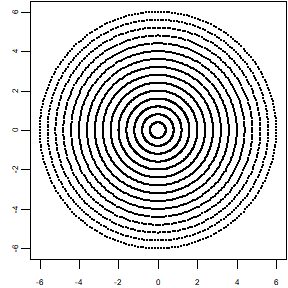## Example 2: Setting basic parameters (number of rings, points per ring, and diameter)

Through the parameters rings and pointsPerRing, the number of rings or mires and the amount of data sampled at each ring can be established, respectively. Besides, the diameter of the resulting dataset can be set with parameter diameter:

  dataset = simulateData(rings = 15, pointsPerRing = 128, diameter = 8)
plot(dataset$x,dataset$y)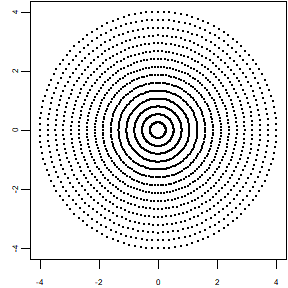## Example 3: Circles with unequally distributed radii

The function allows to change the distribution of circles radii by using the parameter ringRadiiPerturbation. It modifies stochastically the equally distributed radii of the mires between 0 and diameter (by default, diameter=12). Thus, some consecutive pairs of rings are closer (or farther) than others. Typical values of ringRadiiPerturbation would be between 0 (no perturbation) and 1 (high perturbation):

  dataset = simulateData(rings = 15, ringRadiiPerturbation = 0.7)
plot(dataset$x,dataset$y)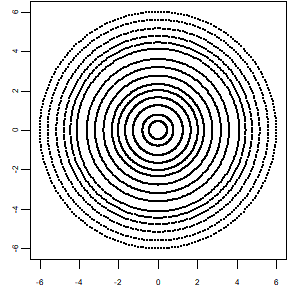## Example 4: Adding mire displacement

In a real measurement, the centers of the mires are not the same, they are off-center. This situation can be also included in the simulation using the parameters maximumMireDisplacement and mireDisplacementAngle. This will produce a pattern of rings in which their centers drift in a straight line. The maximum drift magnitude is given by maximumMireDisplacement (expressed in mm, and should be a reasonable number according to the diameter used). The drift direction is set by mireDisplacementAngle (an angle, expressed in degrees, typically in the range 0-360 with 0 meaning positive x direction). Their use will produce a pattern like this:

  dataset = simulateData(rings = 12, maximumMireDisplacement = 2, mireDisplacementAngle = 45)
plot(dataset$x,dataset$y)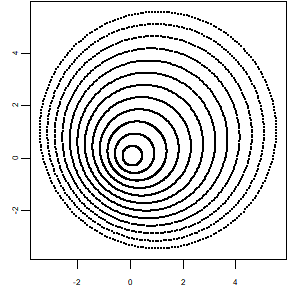In the previous example, the drift magnitude between consecutive rings is constant. In addition, the function allows to perturb this equally-spaced distribution of drifts by setting the parameter mireDisplacementNoise. Typical values of mireDisplacementNoise would be between 0 (no perturbation) and 1 (high perturbation):

  dataset = simulateData(rings = 12, maximumMireDisplacement = 2, mireDisplacementAngle = 45, mireDisplacementPerturbation = 1.2)
plot(dataset$x,dataset$y)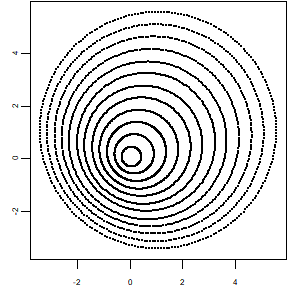Notice that in all the examples so far, the rings keep their perfectly circular shape.

## Example 5: Elliptic mires

Another common measurement pattern is one consisting of elliptic, or nearly elliptic mires. This ellipticity can also be modeled with parameters ellipticAxesRatio and ellipticRotation. The former controls the rate or quotient between the major and minor axes of each ellipse, and the latter controls the orientation of the ellipses (given as an angle, expressed in degrees, typically in the range 0-360 with 0 meaning positive x direction):

  dataset = simulateData(ellipticAxesRatio = 0.8, ellipticRotation = 30)
plot(dataset$x,dataset$y)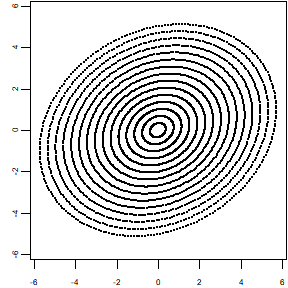## Example 6: Random Cartesian noise

The function simulateData also permits to include random, white noise of a certain magnitude in the Cartesian coordinates of the sampled points. This is done with the parameter overallNoise. Even though this kind of pattern cannot be obtained directly by a Placido-disk topographer (since the points in each mire are sampled equiangularly by the topographer), it might be of interest for some applications.

  dataset = simulateData(overallNoise = 0.2)
plot(dataset$x,dataset$y)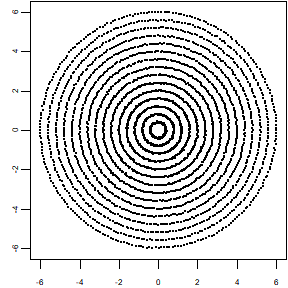## Example 7: Highly irregular pattern, combining different perturbations

Finally, we present an example where most of the previous transformations or perturbations are combined. This will produce a highly irregular pattern, but similar patterns can be found in clinical practice for severe eye diseases:

  dataset = simulateData(maximumMireDisplacement = 1.5, mireDisplacementAngle = 135, ringRadiiPerturbation = 1, mireDisplacementPerturbation = 0.8, ellipticAxesRatio = 0.8, ellipticRotation = 30)
plot(dataset$x,dataset$y)